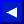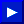## Robert T. Kurosaka

 BYTE Volume 10, issue 3 (March, 1985) Mathematical Recreations: Magic Squares Volume 10, issue 5 (May, 1985) Mathematical Recreations: An Exercise in BASIC Bitwise Logic Operation Volume 10, issue 7 (July, 1985) Mathematical Recreations: Parsing and Solving Linear Equations Volume 10, issue 9 (September, 1985) Mathematical Recreations: π, e, and All That Volume 10, issue 12 (November, 1985) Mathematical Recreations: Repeating Decimals